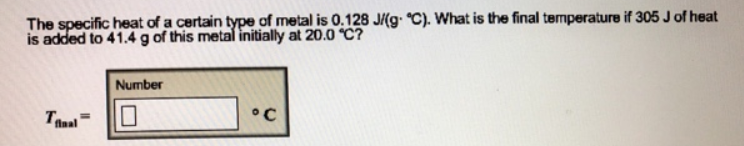Chemistry Calorimetry Solution: The specific heat of a certain type of metal is 0....

# Solution: The specific heat of a certain type of metal is 0.128 J/(g•°C). What is the final temperature if 305 J of heat is added to 41.4 g of this metal initially at 20.0°C?

Problem

The specific heat of a certain type of metal is 0.128 J/(g•°C). What is the final temperature if 305 J of heat is added to 41.4 g of this metal initially at 20.0°C?View Complete Written Solution